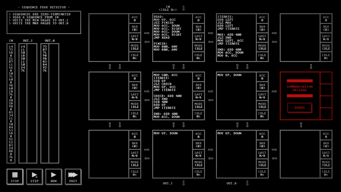## “TIS-100” – SEQUENCE PEAK DETECTOR (SEGMENT 41247)

TIS-100 indexWeird solution here that doesn’t use the `BAK` register at all, but instead does something similar to my NO_BACKUP solution to SEQUENCE COUNTER. I don’t remember why I did this. I’m thinking that I didn’t want to have to send the input to the units that track max and min a third time, or I was running into the instruction limit.

Let’s look at the max unit; the min unit works in essentially the same way, except it compares oppositely and starts with a value of 500 instead of 0.The I/O unit on the left reads values from input and passes them right twice. When it reaches the end of the sequence, instead of passing the 0, it sends a sentinel value of 800 over instead; the maximum value that will appear in a sequence is 99, so it’s a bit overkill.

The max unit on the right keeps the highest value it’s seen in `ACC`, so it starts by subtracting and comparing the input from left (read #1). If the number it was holding was larger, then it adds the input (read #2) to recover the initial value and then restarts.

If we have a negative result instead, then there are two possibilities: the current number in the sequence is larger than the largest one we’ve seen, or we’ve hit the sentinel of 800. We can check which we got by adding 400; if `ACC` is now positive, that means that we had received a normal number, but if it’s still negative, then we got 800. In the former case, we store the input (read #2) in `ACC` as the new max value, and in the latter, we add another 400 to recover the original max value (`ACC` – 800 + 400 + 400 = `ACC`), send that out, and reset the accumulator to 0 for the next sequence.